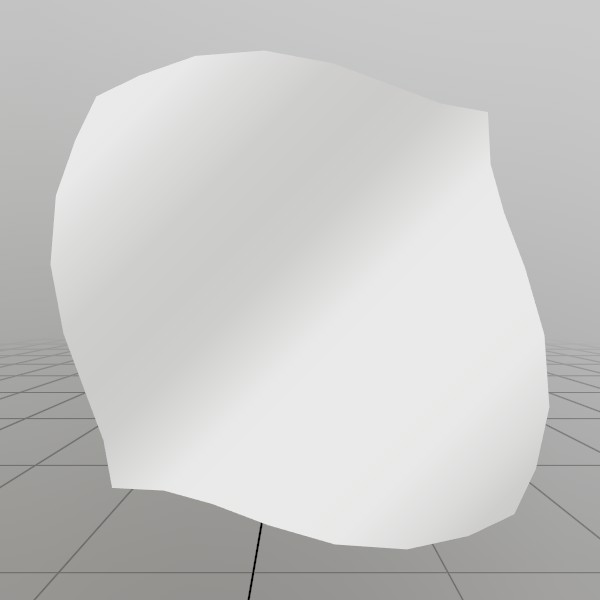# Mesh.SetVerts

``````void SetVerts(Vertex[] verts)
``````

Assigns the vertices for this Mesh! This will create a vertex buffer object on the graphics card right away. If you’re calling this a second time, the buffer will be marked as dynamic and re-allocated. If you’re calling this a third time, the buffer will only re-allocate if the buffer is too small, otherwise it just copies in the data!

Vertex[] verts An array of vertices to add to the mesh. Remember to set all the relevant values! Your material will often show black if the Normals or Colors are left at their default values.

## Examples

### Procedurally generating a wavy gridHere, we’ll generate a grid mesh using Mesh.SetVerts and Mesh.SetInds! This is a common example of creating a grid using code, we’re using a sin wave to make it more visually interesting, but you could also substitute this for something like sampling a heightmap, or a more interesting mathematical formula!

Note: x+y*gridSize is the formula for 2D (x,y) access of a 1D array that represents a grid.

``````const int   gridSize = 8;
const float gridMaxF = gridSize-1;
Vertex[] verts = new Vertex[gridSize*gridSize];
uint  [] inds  = new uint  [gridSize*gridSize*6];

for (int y = 0; y < gridSize; y++) {
for (int x = 0; x < gridSize; x++) {
// Create a vertex on a grid, centered about the origin. The dimensions extends
// from -0.5 to +0.5 on the X and Z axes. The Y value is then sampled from
// a sin wave using the x and y values.
//
// The normal of the vertex is then calculated from the derivative of the Y
// value!
verts[x+y*gridSize] = new Vertex(
new Vec3(
x/gridMaxF-0.5f,
SKMath.Sin((x+y) * 0.7f)*0.1f,
y/gridMaxF-0.5f),
new Vec3(
-SKMath.Cos((x+y) * 0.7f),
1,
-SKMath.Cos((x+y) * 0.7f)).Normalized,
new Vec2(
x / gridMaxF,
y / gridMaxF));

// Create triangle face indices from the current vertex, and the vertices
// on the next row and column! Since there is no 'next' row and column on
// the last row and column, we guard this with a check for size-1.
if (x<gridSize-1 && y<gridSize-1)
{
int ind = (x+y*gridSize)*6;
inds[ind  ] = (uint)((x+1)+(y+1)*gridSize);
inds[ind+1] = (uint)((x+1)+(y  )*gridSize);
inds[ind+2] = (uint)((x  )+(y+1)*gridSize);

inds[ind+3] = (uint)((x  )+(y+1)*gridSize);
inds[ind+4] = (uint)((x+1)+(y  )*gridSize);
inds[ind+5] = (uint)((x  )+(y  )*gridSize);
}
} }
demoProcMesh = new Mesh();
demoProcMesh.SetVerts(verts);
demoProcMesh.SetInds (inds);
``````

Found an issue with these docs, or have some additional questions? Create an Issue on Github!